Want to share your content on R-bloggers? click here if you have a blog, or here if you don't.

I love the formattable package, but I always struggle to remember its syntax. A quick Google search reveals that I’m not alone in this struggle. This post is intended as a reminder for myself of how the package works – and hopefully you’ll find it useful too!

## Default formattable example

The table below is an R data frame (you can turn most things into a data frame using as.data.frame(x), where x is whatever you are converting). It’s by no means as bad as most R tables, but clearly it is not good enough to be shared with others.If we give this table (called prevalence) to formattable, it does a great job just using defaults.

```
library(formattable)
formattable(prevalence)
```## Column alignment

We can control column alignment using the align parameter. In the example below, I set the first column to left-aligned, and the remaining columns are right-aligned.

```
formattable(prevalence, align = c("l", rep("r", NCOL(prevalence) - 1)))
```## Simple column formatting

The main reason people love formattable is the formatting of columns. Below, the first column has been changed to grey, color bars have been added to Average, and the last column has been formatted as percentages. Note that the variable names are surrounded by backticks (the key above your Tab on English-language keyboards), not single quotation marks.

```
prevalence[, "Improvement"] = prevalence[, "Improvement"] / 100
formattable(prevalence,
align = c("l",rep("r", NCOL(prevalence) - 1)),
list(`Indicator Name` = formatter("span", style = ~ style(color = "grey", font.weight = "bold")),
`Average` = color_bar("#FA614B"),
`Improvement` = percent))

```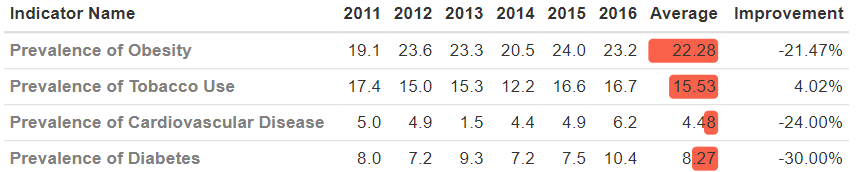## More customized column formatting

In the example above, prior to using formattable I divided the last column by 100, as formattable‘s percent function assumes the inputs are decimals. However, we can perform transformations within formattable. In the code below, I divide by 100 and I also color the values as red or green depending on their value. Note that in the bottom two lines, we define x as being the value by placing it to the left of the ~ and then use it in the function to the right (it is a lambda function, to use some jargon).

```
formattable(prevalence,
align = c("l",rep("r", NCOL(prevalence) - 1)),
list(`Indicator Name` = formatter("span", style = ~ style(color = "grey", font.weight = "bold")),
`Average` = color_bar("#FA614B"),
`Improvement` = formatter("span",
x ~ percent(x / 100),
style = x ~ style(color = ifelse(x&amp;amp;amp;lt; 0, "red", "green")))))
```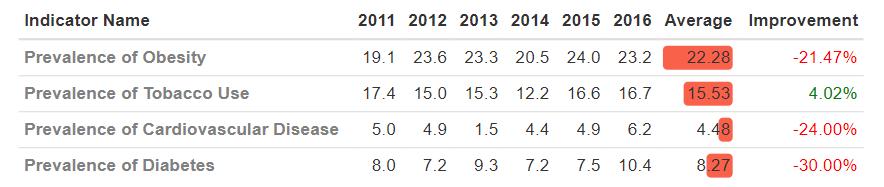Below I extend this even further, replacing the percentages with ticks, crosses, and words.

```
formattable(prevalence,
align = c("l",rep("r", NCOL(prevalence) - 1)),
list(`Indicator Name` = formatter("span", style = ~ style(color = "grey", font.weight = "bold")),
`Average` = color_bar("#FA614B"),
`Improvement` = formatter("span",
x ~ icontext(ifelse(x < 0, "ok", "remove"), ifelse(< 0, "Yes", "No")),
style = x ~ style(color = ifelse(x &amp;amp;amp;lt; 0, "red", "green")))))
```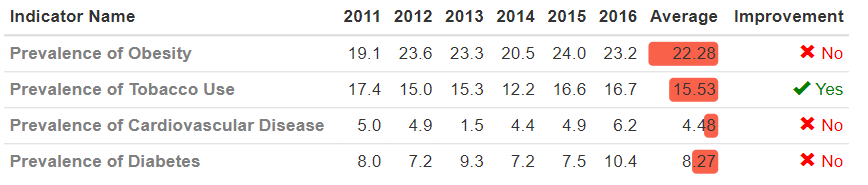## Controlling the width of the bars

In the table below I have used the standard color bar, which scales the bars so that the bar lengths are proportional to the values being displayed. However, IQ cannot really be 0, so arguably the bars are misleading.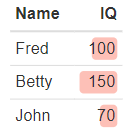The fix to this problem is to provide a function that has a more appropriate mapping between the values and the length of the bars. In the code below, I create a function that returns a 0 for the lowest value (70), and a 1 for the highest value (150).

```
unit.scale = function(x) (x - min(x)) / (max(x) - min(x))
formattable(iq.data,
align = c("l","r"),
list(`IQ` = color_bar("#FA614B66", fun = unit.scale)))
```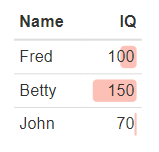## Formatting areas (ranges of cells)

It is possible to also set the shading of ranges of cells,  rather than just individual columns. In the example below, I’ve created a heatmap using two shades of green. In this case I have specified the area using just the columns, but row can also be supplied as well as or in place of col.

```
formattable(prevalence, align = c("l",rep("r", NCOL(prevalence) - 1)), list(
`Indicator Name` = formatter("span", style = ~ style(color = "grey",font.weight = "bold")),
area(col = 2:7) ~ color_tile("#DeF7E9", "#71CA97")))
```In this next example, I first format all the cells to be percentages, and then apply the color shading to the year columns. I have to wrap percent in another function, as percent only works on a single column of numbers.

```
library(formattable)
formattable(prevalence, align = c("l",rep("r", NCOL(prevalence) - 1)),
list(`Indicator Name` = formatter("span", style = ~ style(color = "grey",font.weight = "bold")),
area(col = 2:7) ~ function(x) percent(x / 100, digits = 0),
area(col = 2:7) ~ color_tile("#DeF7E9", "#71CA97")))
```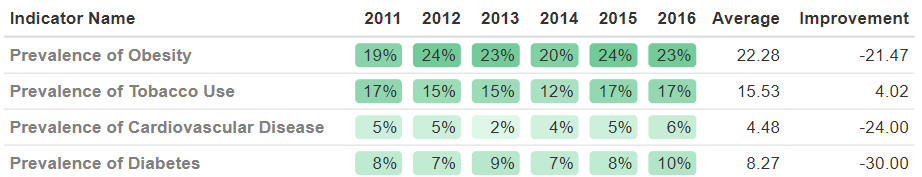## Custom formatters

You can also write your own functions for controlling formatting. In the example below, rather than use formattable‘s in-built color_tile (as done in the previous example), I’ve instead customized it, controlling the padding, border radios, and font color.

```
custom_color_tile &amp;amp;amp;amp;amp;amp;amp;lt;- function (...)
{
formatter("span",
style = function(x) style(display = "block",
`color` = "white",
...))))
}
formattable(prevalence, align = "r", list(
`Indicator Name` = formatter("span", style = ~ style(color = "grey",font.weight = "bold")),
area(col = 2:9) ~ function(x) percent(x / 100, digits = 0),
area(col = 2:7) ~ custom_color_tile("#B1CBEB", "#3E7DCC")))
```

## Arrows (and hiding columns)

Sometimes it is useful to use arrows to show statistical significance. While formattable is not super flexible in this regard, it can do a good job nonetheless. The first step is to create a table where in addition to the data to be displayed, we also have a column containing z-scores.In the code below I first hide the column called z (z = FALSE), add arrows for z-scores of less than -1.96 and greater than 1.96, and make z scores of greater than 0 green and less than 0 red.

```
formattable(prev.sig,
list(z = FALSE,
`2016` = formatter("span",
style = ~ style(color = ifelse(`2016` > `2015`, "green", "red")),
~ icontext(sapply(`z`, function(x) if (x < -1.96) "arrow-down" else if (x > 1.96) "arrow-up" else ""), `2016`))))
```A problem with this table is that the arrows are to the left of the numbers and are not lined up neatly. The only way I have figured out to avoid this is to put the arrows in a separate column, as shown here:This is done by:

• Replacing z with an invisible character ( ).
• Replacing the values with the arrows.
```
names(prev.sig) = " "
formattable(prev.sig,
list( " " = formatter("span",
style = ~ style(color = ifelse(`2016` > `2015`, "green", "red")),
~ icontext(sapply(` `, function(x) if (x < -1.96) "arrow-down" else if (x > 1.96) "arrow-up" else "")))))
```

The sparklines package can be used to create sparklines```
library(sparkline)
sparkline(c(1,2,7,6,5), type = "bar", barColor = "green")
```

We can also include them in formattable tables.The way that we do this is by converting the sparkline into text (character(htmltools::as.tags),  and then (in the last two lines), telling the formattable HTML widget that it also contains sparklines.

```
library(sparkline)
library(formattable)
df = data.frame("Type" = c("bar", "line", "bullet", "pie", "tristate", "discrete"),
Sparkline = c(as.character(htmltools::as.tags(sparkline(c(1,2,7,6,5), type = "bar"))),
as.character(htmltools::as.tags(sparkline(c(1,2,7,6,5), type = "line"))),
as.character(htmltools::as.tags(sparkline(c(1,2,7,6,5), type = "bullet"))),
as.character(htmltools::as.tags(sparkline(c(1,2,7,6,5), type = "pie"))),
as.character(htmltools::as.tags(sparkline(c(-1,0,1,1,1,-1,0,2), type = "tristate"))),
as.character(htmltools::as.tags(sparkline(c(1,2,7,6,5), type = "discrete")))))
out = as.htmlwidget(formattable(df))
out\$dependencies = c(out\$dependencies, htmlwidgets:::widget_dependencies("sparkline", "sparkline"))
out
```

## Putting it all together

In this final example, I combine many of the different ideas I’ve discussed into one table.```
library(formattable)
library(sparkline)
prevalence\$` ` = c(4.1, -.3, .5, 1.4)
prevalence\$`2012` = apply(prevalence[, 2:7], 1, FUN = function(x) as.character(htmltools::as.tags(sparkline(as.numeric(x), type = "line"))))
names(prevalence) = "  "
new.prevalance = prevalence[, c(1, 2, 3, 7, 10)]
out = as.htmlwidget(formattable(new.prevalance,
align = c("l",rep("r", NCOL(prevalence) - 1)),
list(`Indicator Name` = formatter("span", style = ~ style(color = "grey", font.weight = "bold")),
" " = formatter("span",
style = ~ style(color = ifelse(`2016` > `2011`, "green", "red")),
~ icontext(sapply(` `, function(x) if (x < -1.96) "arrow-down" else if (x> 1.96) "arrow-up" else ""))))))
out\$dependencies = c(out\$dependencies, htmlwidgets:::widget_dependencies("sparkline", "sparkline"))
out
```

## View all the source code and play around with these examples yourself

I’ve created all the examples in this post in a live Displayr document, so you can look at the code and play around with it yourself. Click here to view the code and tables discussed in this post.

## Acknowledgements

The main example and many of the ideas in this post are from LITTLE MISS DATA, although I’ve reworked the code quite significantly. The hack for getting sparklines into the tables comes from HTML widget guru Kent RussellBert Wassink provided the trick for having a blank column name. My colleague Justin helped me a lot with this post.

View and modify the code in all these examples here!